## Resource of the week: Year 4 pictogram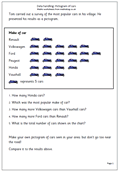Graphs are often perceived as a quite easy part of maths, but many children find interpreting them correctly quite tricky. This page looks at the most popular cars seen in a village. Each picture of a car represents 5 cars. Again this page should be seen more as a starter to go and collect data themselves and make their own pictograms.  Problems might arise when the data is not in whole fives or tens. This can be solved by showing part of a car eg a wheel could represent one car. This would need to be shown clearly on the pictogram.

This and other handling Data activities can be found in our Year 4 resources.

Pictogram: most popular cars

## What are Dyscalculia and Dyslexia?Dyscalculia and Dyslexia! Two tricky words to spell, but they could be part of the reason why your child has turned off maths, even at an early age.

Sometimes the problems our children have with maths seem almost inexplicable and it can be very  frustrating as an adult trying to help a child who just seems ‘not to get maths!’ The real causes of problems may be quite deep rooted and have little or nothing to do with intelligence or lack of effort, but have much more to do with processing and acquiring the skills of mathematics. URBrainy have just published the first of a short series of simple, straightforward articles on how dyslexia and dyscalculia can affect children’s progress in maths.

If you have a child who has problems with time or direction, reverses digits when writing numbers down, or can’t seem to remember simple sequences or ‘tables’ then it would be well worth taking a couple of minutes to read this first article.

Read the article: What are Dyscalculia and Dyslexia?

## Calculator game: 5x table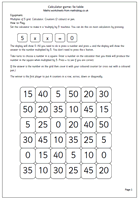This is another in our series of using a calculator to help learn and reinforce tables. To be successful with this game you need to know your 5x table: the calculator just confirms your correct mental arithmetic (or not!). This series of calculator activities for 2x, 3x, 5x and 10x tables are all found in the Year 3: Know number facts section.

## Free maths worksheet: Counting in steps of 25 and 50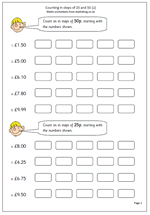By year 4 children should be confident enough to count on in steps of 50 or 25. This can be useful when using money or length. Here we have a straightforward free maths worksheet that looks at counting in these steps. Some of the counting on in fifties start with multiples of 50 but the later questions don’t, but children should quickly see the patterns involved.

Counting in steps of 50 or 25_(1)

## Coming soon: counting, calculator game and dividing decimalsA mixed bag of activities coming next week.

By year 4 children should be confident enough to count on in steps of 50 or 25 and we will be publishing a practice sheet on this as it is seldom found elsewhere, but does come into the Year 4 objectives.

We will also be publishing another in our series of using a calculator to help learn and reinforce tables. To be successful with this game you need to know your 5x table: the calculator just confirms your correct mental arithmetic (or not!). Suitable for Year 3 upwards.

Also coming soon is a good revision sheet for Year 6 to make sure that they understand the process of dividing decimals by 10 or 100. Knowledge of this is crucial for success in High School.

## Maths worksheet: Multiply decimals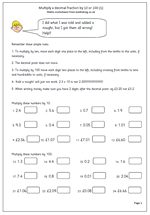There are some definite dos and don’ts when it comes to multiplying decimals ‘in your head’.

To multiply by 10 move each digit one place to the left, including tenths to the units, if necessary.

To multiply by 100 move each digit two places to the right.

The big don’t is to think that ‘add a nought’ is a short cut to multiplying by 10. 2.3 x 10 is not 2.30!!

This is a good check up page to make sure children understand what they are doing.

Multiply decimal fractions by 10 and 100 (1)

## Resource of the week: Tables square and square numbers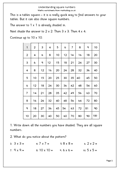This worksheet has a 10×10 multiplication square which is a brilliant aid to helping with tables, but it is also excellent for showing the pattern of square numbers from 1 to 10.

A square number is made by multiplying a whole number by itself. eg 4 x 4 = 16.

16 is a square number.

It is quite useful to learn the first 10 square numbers off by heart:

1, 4, 9, 16, 25, 36, 49, 64, 81, 100.

We will look more closely at square numbers later.

Square numbers (pg 1)

## Resource of the week: Equivalent fractions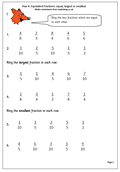Once the idea of equivalent fractions has been understood it becomes possible to compare two fractions to see which is the larger. The first thing to remember is that the larger the number on the bottom of the fraction, the smaller each part of the fraction is. So 4/100 is much smaller than 4/25.

Now this is also easy if the bottom number (denominator) is the same in each fraction eg 1/5 is smaller than 3/5.

The difficulty comes when the numbers are not the same. How do you compare 3/5 with 7/10?

The easiest way is to make the bottom number of each fraction the same, and in the case above this will mean converting the 3/5 into tenths. We can do this by multiplying the 5 by 2 to make 10 and we have to do the same with the top number, multiplying the 3 by 2. In this way 3/5 can be converted to 6/10.

3/5 and 6/10 are equal, or equivalent.

It is now easy to see that 6/10 is smaller than 7/10, so 3/5 must also be smaller than 7/10. Job done!

Later it will become harder to convert so that the denominators are the same; sometimes you have to multiply both fractions by different numbers, but this comes later! At the moment it is important to get the basics correct.

Equivalent fractions: comparing

## Maths worksheet: Subtraction of money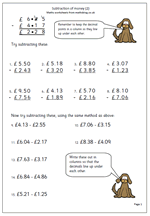Using written methods of subtraction can prove quite tricky with money as it involves decimals. It is important to lay the question out in the standard way, even if it is shown horizontally or just as a written problem and most importantly, to keep the decimal points in a line.

Watch out when there is a zero on the top line as many children go for the easy option and say ‘nought take away 3 is 3’ rather than going through the carrying process.

This is the second in our series on subtracting money.

Standard money subtraction_p2

## Counting back in tens: Year 3 revision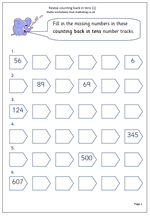In year 3 many children still find it tricky to count on and back and there is a danger that they are moved on to harder maths before they have mastered this basic skill. This is a page which can be used as a check to make sure that they are confident with counting back in tens from any 2 or 3-digit number.

Revise counting back in tens